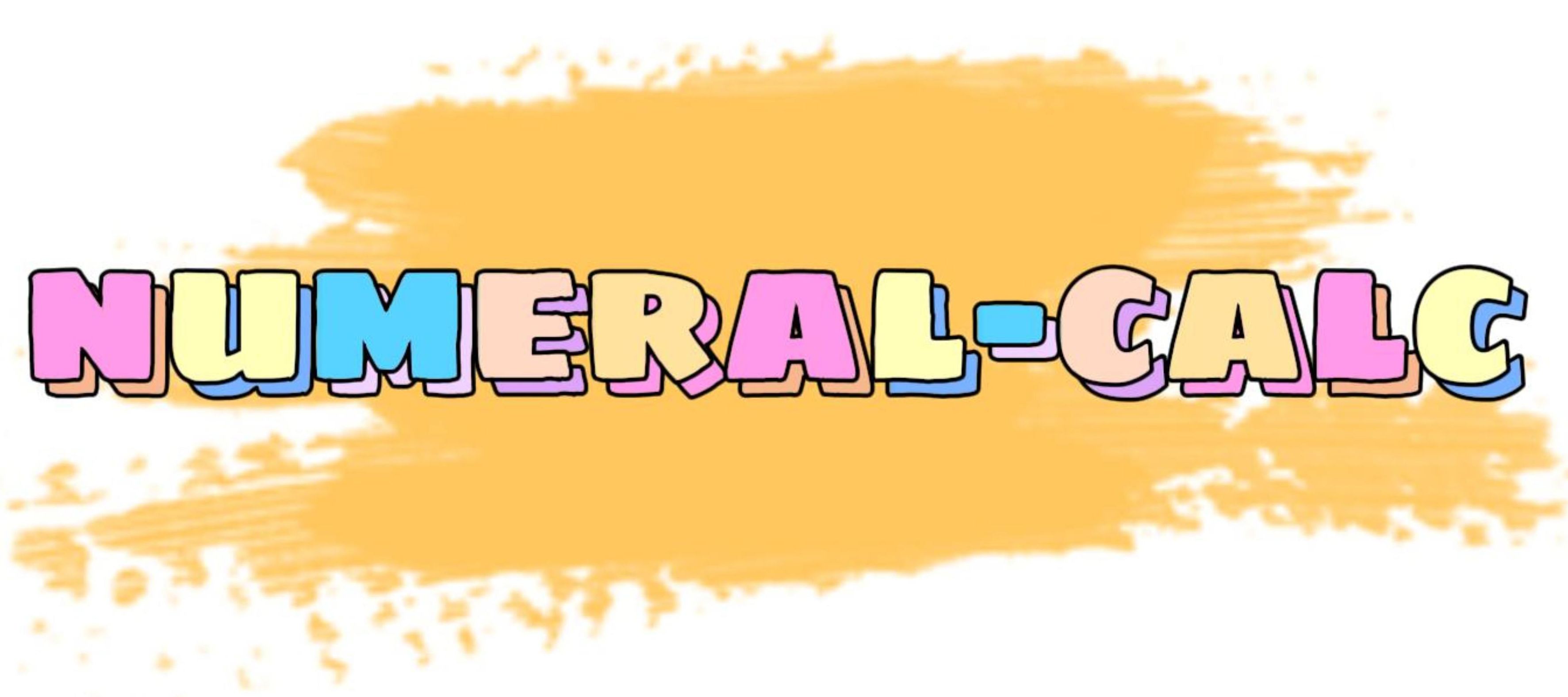# npm

## numeral-calc1.0.9 • Public • Published

# numeral-calcSolve the ECMAScript language large number calculation and floating point number problem.

Supports scientific numeration calculations.

## Installation

The numeral-calc package lives in npm. To install the latest stable version, run the following command:

`npm i numeral-calc`

Or if you're using yarn:

`yarn add numeral-calc`

## Usage

Codepen Demo

```import { add, sub, multi, divide, calc } from 'numeral-calc';

In the same way: calc(0.1,0.2,"+")  //  "+" can be replaced by "and" or "plus" or "add"

sub(0.3,0.1) // "0.2"
In the same way: calc(0.3,0.1,"-")  //  "-" can be replaced by "minus" or "sub" or "subtract"
/* Support for custom scientific notation. */
sub(1.02e1,1.3e-4)  //  "10.19987"

multi(1.15,100) // "115"

multi(1.15,100,2) // "230"
In the same way: calc(1.15,100,"*")   //  "*" can be replaced by "x" or "multi" or "multiply"

divide(9007199254740997,2) // "4503599627370498.5"
In the same way: calc(9007199254740997,2,"/")   //  "/" can be replaced by "divide"```

## Objective

Use the simplest method to solve the most fundamental problem.

• No new types are generated.
• Floating-point and large numbers are handled together using numeral-calc.

The underlying method relies on the BigInt data type.

Addition and multiplication can take multiple arguments.

## Notice

There seems to be no such business scenario.

• The divisor cannot exceed the safe range of the JS language.
• Scientific notation exponentials cannot exceed the safe limits of the JS language.

## Keywords

### Install

`npm i numeral-calc`

### Repository

github.com/NickLJudy/numeral-calc

40

1.0.9